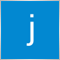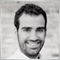# Discussion of article "Introduction to MQL5: How to write simple Expert Advisor and Custom Indicator" - page 216

Ibrahim Melssen:
I have copy paste the Expert Advisor and try to test it with Strategytester. But it doesn't make any trades. I am new to MQL5 and programming so maybe I just made a stupid mistake. It compiled without any errors. I'd really like the strategy! Anyone ideas why it doesn't run on strategytester..?

Same here men I cant seem to find out why65

Guin:

Im new at mql5 programming

Was trying to learn through this example, but im a bit lost with the loop at the end of the indicator build. Where exactly did he assign a value to day_n variable?

Because the loop will check for day_n<day_t. How can the program know day_n value?

And how is it calculated at all? Lets assume rate_total = 10 and there is still no calculated bar yet. So prev_calculated = 0

day_t=time (TODAY! since its counted backwards)/PeriodSeconds... since it starts counting from 1970, lets assume it starts count from 10 days ago. so it should give 10, right?

So day_t=10. Now it checks if dayt > dayn. I dont know dayn, but i know dayt=10. Im gonna assume dayn is zero, since there is no value.

Then dayn becomes 10 too. Ok.

Second roll of the loop. prev_calculated + 1= 1.

DayT=time (yesterday)/period... remember, it starts counting from 10 days ago... but now only until yesterday. it should give a value of 9, right?

but now dayN < dayT is false. Then it starts to perform the else expressions. Ok. I understand.

It then will calculate all the bu[] and bd[]. Ok. The loop will end when prev < total rate is false.

But when a new bar arrives, and it becomes true again, i will start from zero again? Or it will start from 10 and go straight to the else part?

Thanks!!!!

You need to divide into two parts:

1 At the first time indicator applied to the chart:  prev_calculated =0 , i = 0, i++  until  i = rates_total,  it gets out of loop    (time is from the past not present.)

2 New bar is started:  prev_calculated will be less than rates_total so condition is true,  the loop will run only on that new bar9

Hi @Guin,

I don't think your question has been answered correctly yet. If you've used the code in the example, you probably end up with an invisible indicator that doesn't make any sense and you can't see on the chart. That's because the code never goes through this block:

```if(day_n < day_t){
day_n = day_t;
h_day = high[i];
l_day = low[i]
}```

The reason is that day_n is not manually set to any default value, and the comparison day_n < day_t will always result in false. According to the debugger, the value of day_n when not explicitly set is "2076449103".

Just change the definition of day_n to something like:

`int day_n = 0, day_t;`

I hope that helps.

Guin:

Im new at mql5 programming

Was trying to learn through this example, but im a bit lost with the loop at the end of the indicator build. Where exactly did he assign a value to day_n variable?

Because the loop will check for day_n<day_t. How can the program know day_n value?

Files: